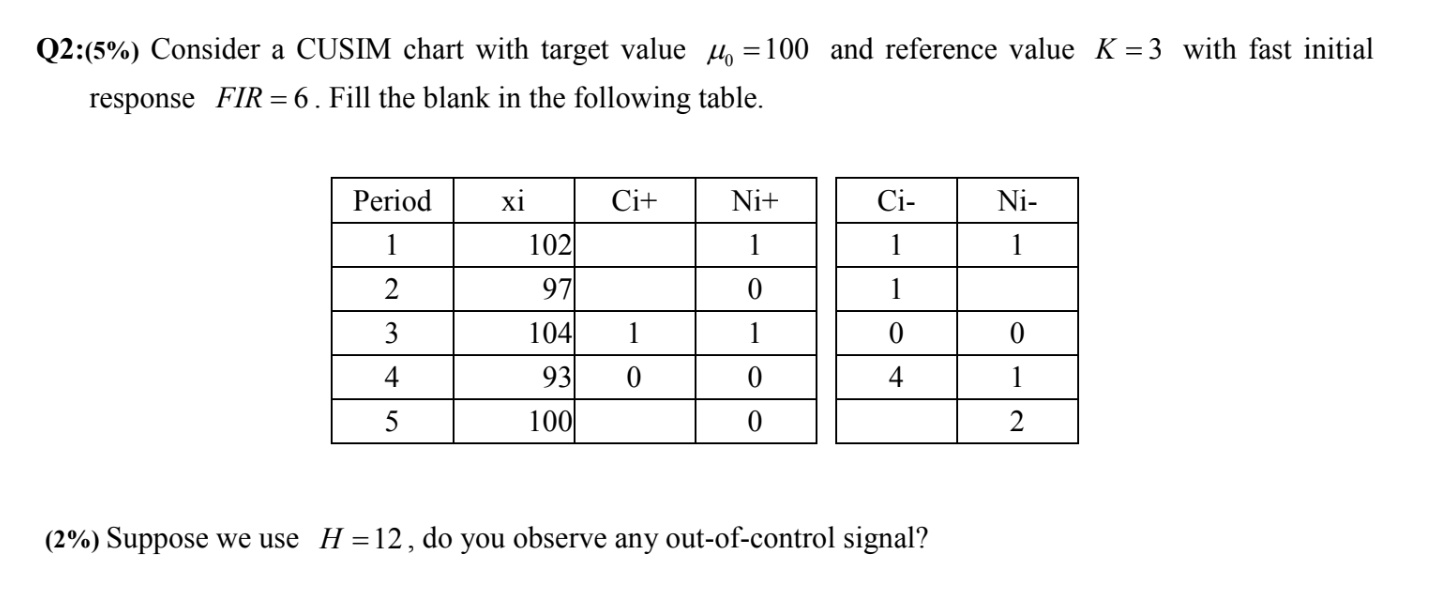Home / Expert Answers / Operations Management / q2-5-consider-a-cusim-chart-with-target-value-mu-0-100-and-reference-value-k-3-pa568

# (Solved): Q2:(5\%) Consider a CUSIM chart with target value $$\mu_{0}=100$$ and reference value $$K=3$$ ...Q2:(5\%) Consider a CUSIM chart with target value $$\mu_{0}=100$$ and reference value $$K=3$$ with fast initial response $$F I R=6$$. Fill the blank in the following table. (2\%) Suppose we use $$H=12$$, do you observe any out-of-control signal?

We have an Answer from Expert